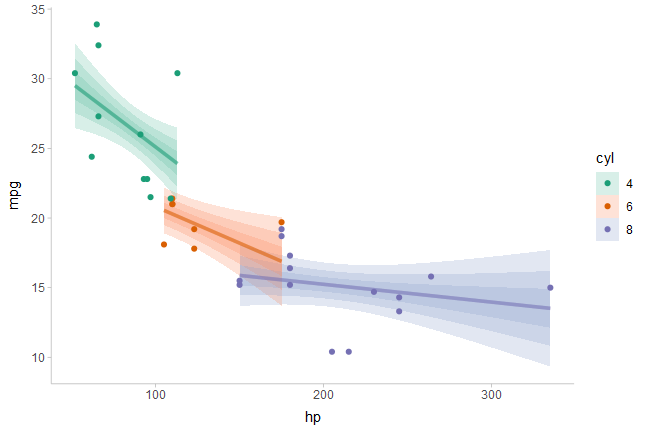# Frequentist uncertainty visualization

## Introduction

This vignette shows how to combine the ggdist geoms with output from the broom package to enable visualization of uncertainty from frequentist models. The general idea is to use the stat_dist_... family of ggplot stats to visualize confidence distributions instead of visualizing posterior distributions as we might from a Bayesian model. For more information on that family of stats and geoms, see vignette("slabinterval").

Confidence distributions are a way of unifying the notion of sampling distributions, bootstrap distributions, and several other concepts in frequentist inference. They are a convenient tool for visualizing uncertainty in a way that generalizes across Bayesian and frequentist frameworks: where in a Bayesian framework we might visualize a probability distribution, in the frequentist framework we visualize a confidence distribution. This gives us a way to use the same geometries for uncertainty visualization in either framework.

For more on confidence distributions, see: Xie, Min‐ge, and Kesar Singh. Confidence distribution, the frequentist distribution estimator of a parameter: A review. International Statistical Review 81.1 (2013): 3-39.

## Setup

The following libraries are required to run this vignette:

library(dplyr)
library(tidyr)
library(ggdist)
library(ggplot2)
library(broom)
library(modelr)

theme_set(theme_ggdist())

## Point estimates

We’ll start with an ordinary least squares (OLS) linear regression analysis of this simple dataset:

set.seed(5)
n = 10
n_condition = 5
ABC =
tibble(
condition = rep(c("A","B","C","D","E"), n),
response = rnorm(n * 5, c(0,1,2,1,-1), 0.5)
)

This is a typical tidy format data frame: one observation per row. Graphically:

ABC %>%
ggplot(aes(x = response, y = condition)) +
geom_point(alpha = 0.5) +
ylab("condition")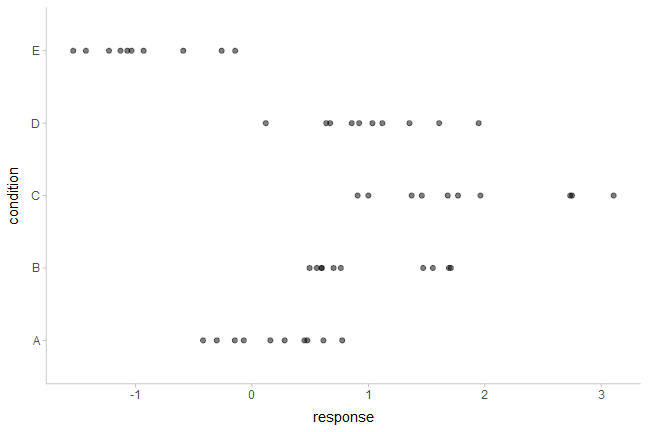And a simple linear regression of the data is fit as follows:

m_ABC = lm(response ~ condition, data = ABC)

The default summary is not great from an uncertainty communication perspective:

summary(m_ABC)
##
## Call:
## lm(formula = response ~ condition, data = ABC)
##
## Residuals:
##     Min      1Q  Median      3Q     Max
## -0.9666 -0.4084 -0.1053  0.4104  1.2331
##
## Coefficients:
##             Estimate Std. Error t value Pr(>|t|)
## (Intercept)   0.1816     0.1732   1.048  0.30015
## conditionB    0.8326     0.2450   3.399  0.00143 **
## conditionC    1.6930     0.2450   6.910 1.38e-08 ***
## conditionD    0.8456     0.2450   3.452  0.00122 **
## conditionE   -1.1168     0.2450  -4.559 3.94e-05 ***
## ---
## Signif. codes:  0 '***' 0.001 '**' 0.01 '*' 0.05 '.' 0.1 ' ' 1
##
## Residual standard error: 0.5478 on 45 degrees of freedom
## Multiple R-squared:  0.7694, Adjusted R-squared:  0.7489
## F-statistic: 37.53 on 4 and 45 DF,  p-value: 8.472e-14

So let’s try half-eye plots instead. The basic idea is that we need to get the three parameters for the sampling distribution of each parameter and then use stat_dist_halfeye() to plot them. The confidence distribution for parameter $$i$$, $$\tilde\beta_i$$, from an lm model is a scaled-and-shifted t distribution:

$\tilde\beta_i \sim \textrm{student_t}\left(\nu, \hat\beta_i, \sigma_{\hat\beta_i}\right)$

With:

• $$\nu$$: degrees of freedom, equal to df.residual(m_ABC)
• $$\hat\beta_i$$: location, equal to the point estimate of the parameter (estimate column from broom::tidy())
• $$\sigma_{\hat\beta_i}$$: scale, equal to the standard error of the parameter estimate (std.error column from broom::tidy())

We can get the estimates and standard errors easily by using broom::tidy():

tidy(m_ABC)
term estimate std.error statistic p.value
(Intercept) 0.1815842 0.1732360 1.048190 0.3001485
conditionB 0.8326303 0.2449927 3.398593 0.0014276
conditionC 1.6929997 0.2449927 6.910410 0.0000000
conditionD 0.8455952 0.2449927 3.451513 0.0012237
conditionE -1.1168101 0.2449927 -4.558545 0.0000394

Putting everything together, we have:

m_ABC %>%
tidy() %>%
ggplot(aes(y = term)) +
stat_dist_halfeye(
aes(dist = "student_t", arg1 = df.residual(m_ABC), arg2 = estimate, arg3 = std.error)
)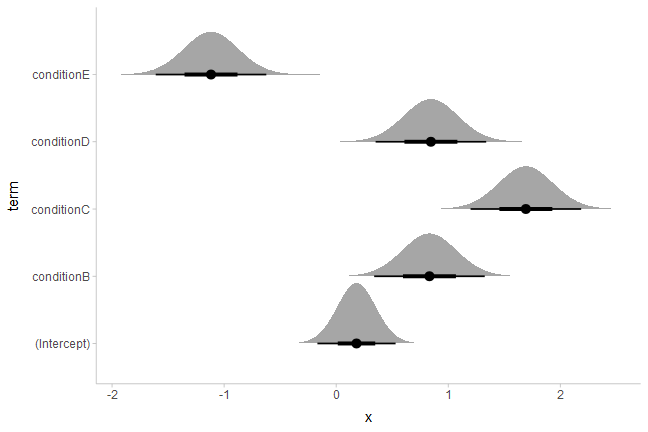If we would rather see uncertainty in conditional means, we can instead use modelr::data_grid() along with broom::augment() (similar to how we can use modelr::data_grid() with tidybayes::add_fitted_draws() for Bayesian models). Here we want the confidence distribution for the mean in condition $$c$$, $$\tilde\mu_c$$:

$\tilde\mu_c \sim \textrm{student_t}\left(\nu, \hat\mu_c, \sigma_{\hat\mu_c} \right)$

With:

• $$\nu$$: degrees of freedom, equal to df.residual(m_ABC)
• $$\hat\mu_c$$: location, equal to the point estimate of the mean in condition $$c$$ (.fitted column from broom::augment())
• $$\sigma_{\hat\mu_c}$$: scale, equal to the standard error of the mean in condition $$c$$ (.se.fit column from broom::augment(..., se_fit = TRUE))

Putting everything together, we have:

ABC %>%
data_grid(condition) %>%
augment(m_ABC, newdata = ., se_fit = TRUE) %>%
ggplot(aes(y = condition)) +
stat_dist_halfeye(
aes(dist = "student_t", arg1 = df.residual(m_ABC), arg2 = .fitted, arg3 = .se.fit),
scale = .5
) +
# we'll add the data back in too (scale = .5 above adjusts the halfeye height so
# that the data fit in as well)
geom_point(aes(x = response), data = ABC, pch = "|", size = 2, position = position_nudge(y = -.15))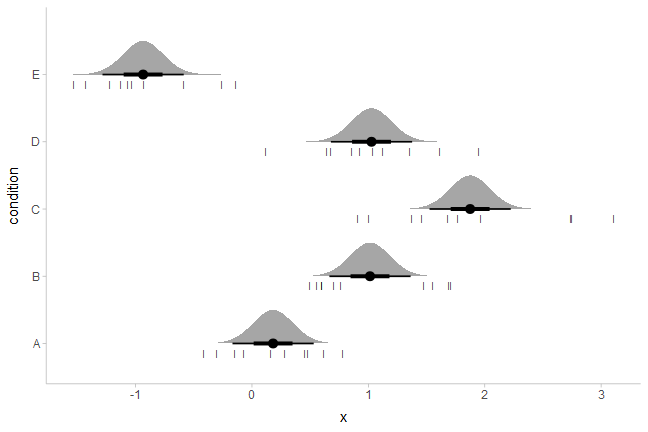Of course, this works with the entire stat_dist_... family. Here are gradient plots instead:

ABC %>%
data_grid(condition) %>%
augment(m_ABC, newdata = ., se_fit = TRUE) %>%
ggplot(aes(y = condition)) +
aes(dist = "student_t", arg1 = df.residual(m_ABC), arg2 = .fitted, arg3 = .se.fit),
scale = .5
)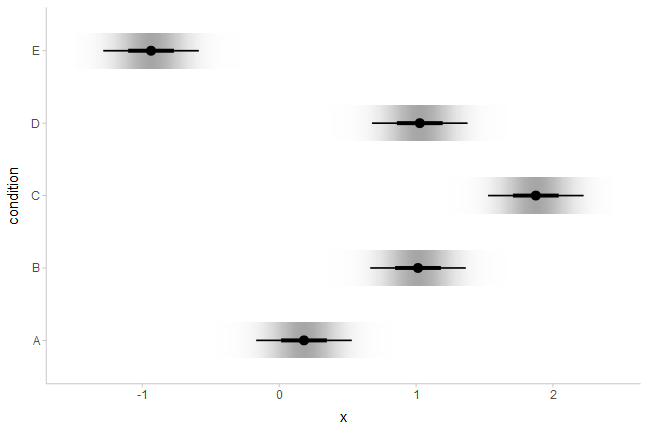Or complementary cumulative distribution function (CCDF) bar plots:

ABC %>%
data_grid(condition) %>%
augment(m_ABC, newdata = ., se_fit = TRUE) %>%
ggplot(aes(y = condition)) +
stat_dist_ccdfinterval(
aes(dist = "student_t", arg1 = df.residual(m_ABC), arg2 = .fitted, arg3 = .se.fit)
)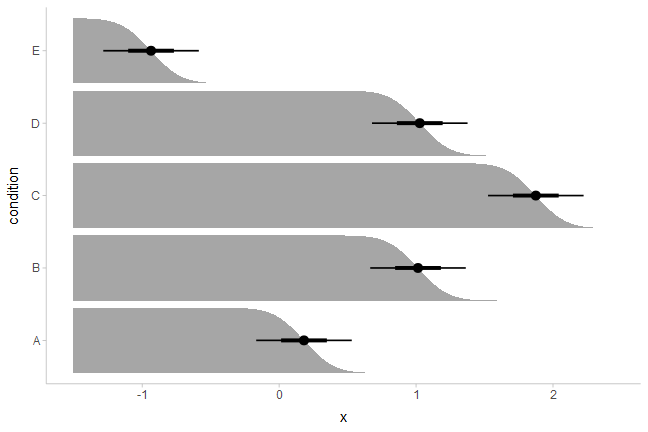We can also create quantile dotplots by using the dots family of geoms. Quantile dotplots show quantiles from a distribution (in this case, the sampling distribution), employing a frequency framing approach to uncertainty communication that can be easier for people to interpret (Kay et al. 2016, Fernandes et al. 2018):

ABC %>%
data_grid(condition) %>%
augment(m_ABC, newdata = ., se_fit = TRUE) %>%
ggplot(aes(y = condition)) +
stat_dist_dots(
quantiles = 100,
aes(dist = "student_t", arg1 = df.residual(m_ABC), arg2 = .fitted, arg3 = .se.fit)
)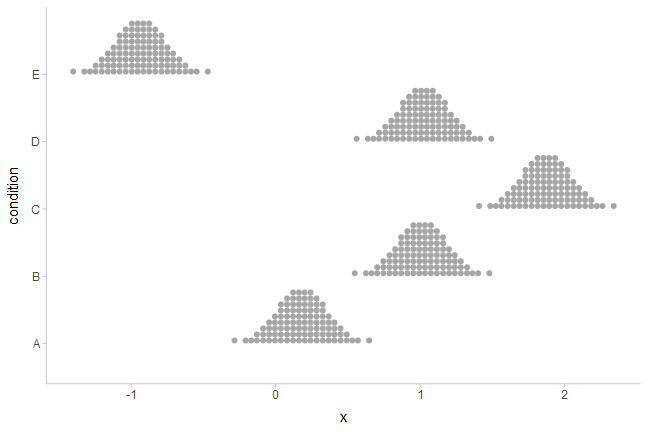See vignette("slabinterval") for more examples of uncertainty geoms and stats in the slabinterval family.

## For a fit line

The same principle of reconstructing the confidence distribution allows us to use stat_dist_lineribbon() to construct uncertainty bands around regression fit lines. Here we’ll reconstruct an example with the mtcars dataset from vignette("tidy-brms", package = "tidybayes"), but using lm() instead:

m_mpg = lm(mpg ~ hp * cyl, data = mtcars)

Again we’ll use modelr::data_grid() with broom::tidy(), but now we’ll employ stat_dist_lineribbon():

mtcars %>%
group_by(cyl) %>%
data_grid(hp = seq_range(hp, n = 101)) %>%
augment(m_mpg, newdata = ., se_fit = TRUE) %>%
ggplot(aes(x = hp, fill = ordered(cyl), color = ordered(cyl))) +
stat_dist_lineribbon(
aes(dist = "student_t", arg1 = df.residual(m_mpg), arg2 = .fitted, arg3 = .se.fit),
alpha = 1/4
) +
geom_point(aes(y = mpg), data = mtcars) +

scale_fill_brewer(palette = "Set2") +
scale_color_brewer(palette = "Dark2") +
labs(
color = "cyl",
fill = "cyl",
y = "mpg"
)